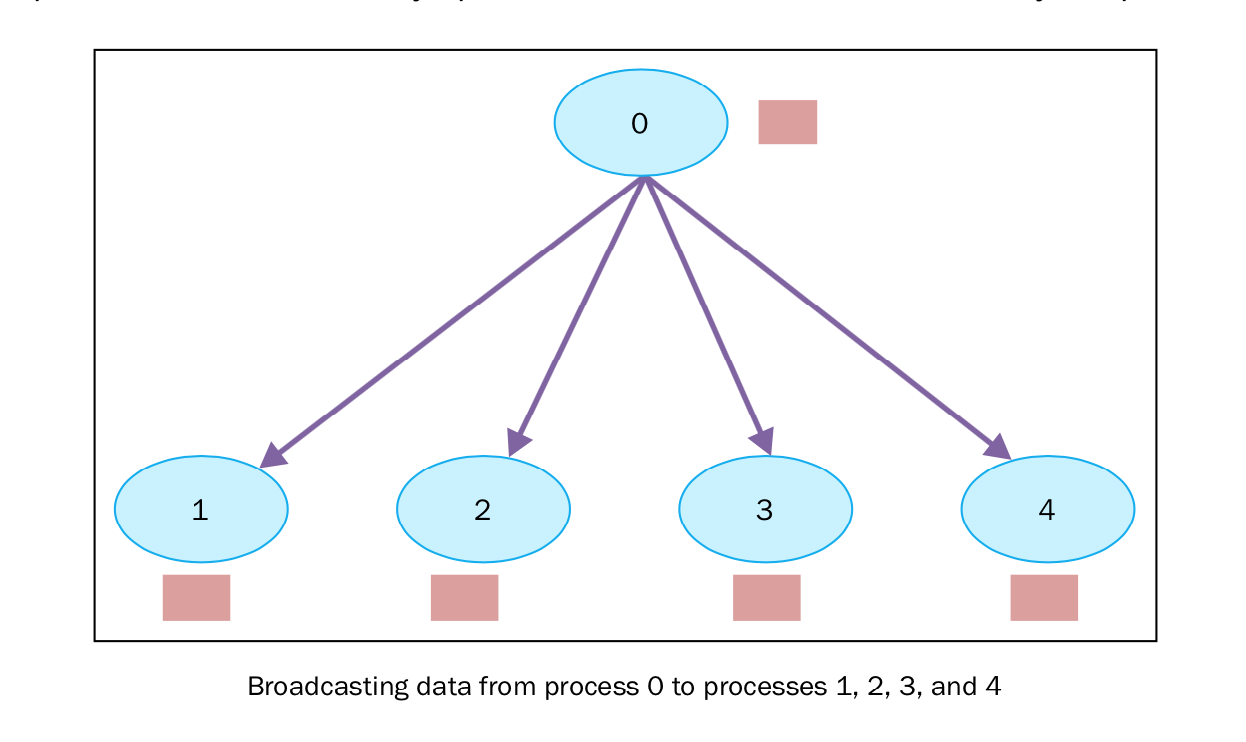```buf = comm.bcast(data_to_share, rank_of_root_process)
```

## 14.1. 如何做…¶

```from mpi4py import MPI
comm = MPI.COMM_WORLD
rank = comm.Get_rank()
if rank == 0:
variable_to_share = 100
else:
variable_to_share = None
variable_to_share = comm.bcast(variable_to_share, root=0)
print("process = %d" %rank + " variable shared  = %d " %variable_to_share)
```

```C:\>mpiexec -n 10 python broadcast.py
process = 0 variable shared  = 100
process = 8 variable shared  = 100
process = 2 variable shared  = 100
process = 3 variable shared  = 100
process = 4 variable shared  = 100
process = 5 variable shared  = 100
process = 9 variable shared  = 100
process = 6 variable shared  = 100
process = 1 variable shared  = 100
process = 7 variable shared  = 100
```

## 14.2. 讨论¶

`rank` 等于0的root进程初始化了一个变量， `variable_to_share` ，值为100.这个变量将通过通讯组发送给其他进程。:

```if rank == 0:
variable_to_share = 100
```

```variable_to_share = comm.bcast(variable_to_share, root=0)
```

```print("process = %d" %rank + " variable shared  = %d " %variable_to_share)
```

## 14.3. 了解更多¶

1. 组中的进程提供通讯的屏障
2. 通讯方式包括：
• 将一个进程的数据广播到组中其他进程中
• 从其他进程收集数据发给一个进程
• 从一个进程散播数据散播到其他进程中
3. 减少操作